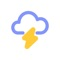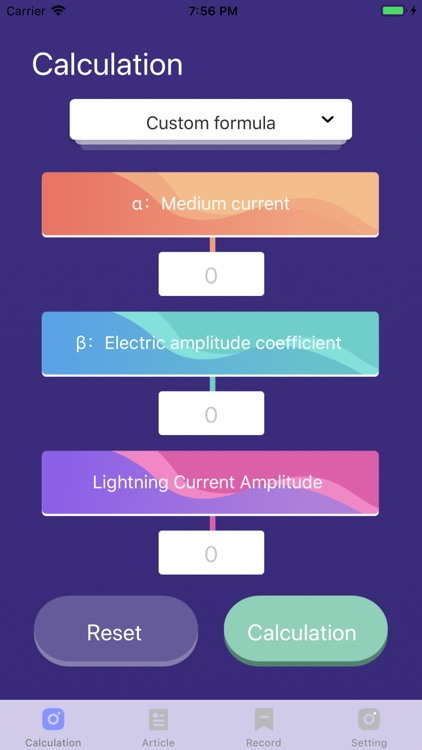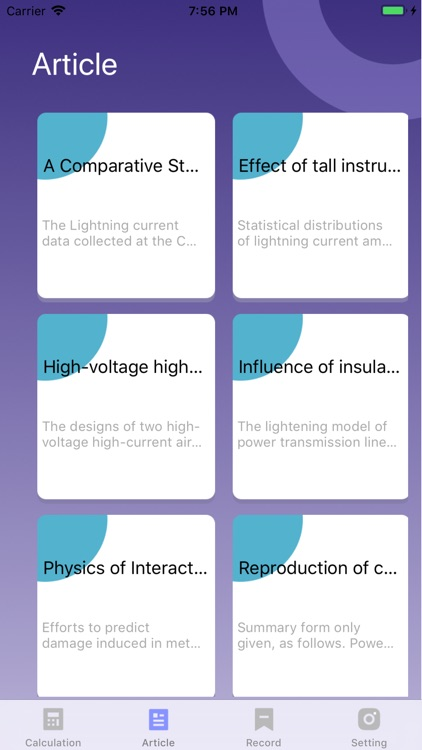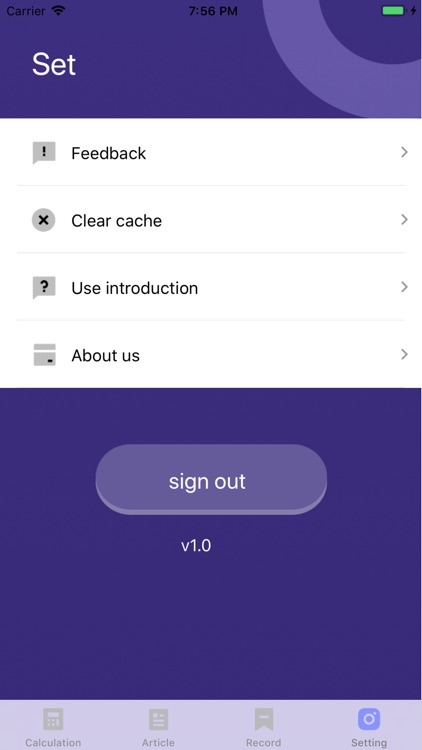## The APP designed for grid engineers, it helps grid engineers quickly calculate probability of lightning current amplitude# lightning amplitude

by Francisco McEwanThe APP designed for grid engineers, it helps grid engineers quickly calculate probability of lightning current amplitude. Engineers can choose the calculation formula that suits their needs according to their needs, and further calculate the lightning flashover rate based on the calculation result.### App Details

Version
1.0
Rating
NA
Size
17Mb
Genre
Productivity Utilities
Last updated
July 10, 2019
Release date
July 10, 2019

### App Store Description

The APP designed for grid engineers, it helps grid engineers quickly calculate probability of lightning current amplitude. Engineers can choose the calculation formula that suits their needs according to their needs, and further calculate the lightning flashover rate based on the calculation result.

In the accidents of the power system, the faults of the transmission lines account for the majority, and the faults of the high-voltage transmission lines are mainly caused by lightning strikes. Lightning flashover rate is an important indicator to evaluate the performance of lightning strikes. The accuracy of calculation is especially important!

The lightning current amplitude probability is one of the important parameters for calculating the lightning flashover rate. There are many calculation methods for this parameter, and the calculation procedure is complicated. However, the accuracy of the calculation results of probability of lightning current amplitude is very important, which will affect the accuracy of the grid engineer in lightning protection calculation and the effectiveness of the lightning protection scheme.

[Instructions]: